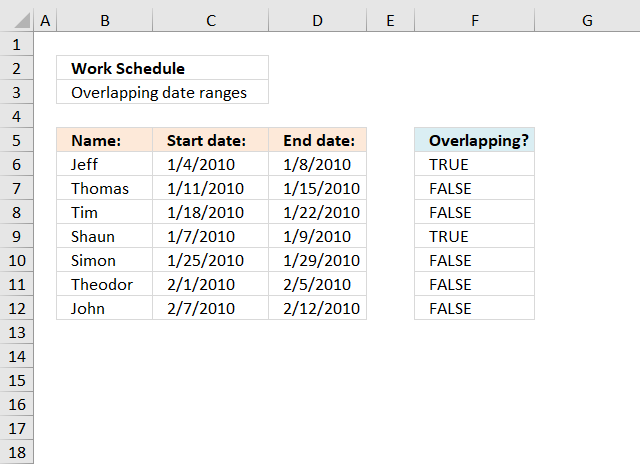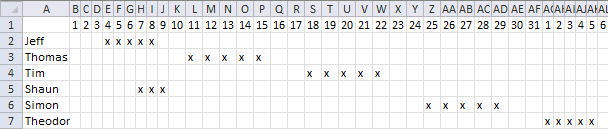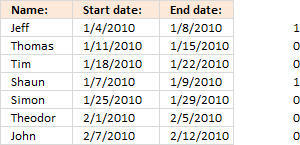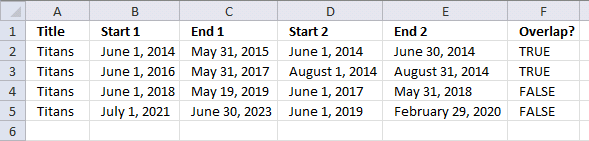Author: Oscar Cronquist Article last updated on October 31, 2018The formula in cell F6 returns TRUE if the date range on the same row overlaps another date range in cell range C6:D12.

Formula in cell F6:

=SUMPRODUCT((C6<=\$D\$6:\$D\$12)*(D6>=\$C\$6:\$C\$12))>1

Copy cell E6 and paste it down as far as needed.

If there are multiple overlapping date range it can be quite hard to identify which date range overlaps, I made an article that assists you in finding overlapping date ranges.

There is also a formula for counting overlapping days: Counting overlapping days

Here is a picture of the date ranges. Jeff's and Shaun's date ranges overlap.If you are interested in a formula that creates a schedule based on date ranges, like the one above, read the following article: Visualize date ranges in a calendar

Explaining formula in cell F6

I am using date values in this worksheet and Excel handles dates as numbers if you don't know that. For example, 1/1/1900 is 1. 1/1/2018 is 43101.

You can verify this by typing 1/1/1900 in a cell and then press Enter. Select the cell containing the date and press and hold CTRL, then press 1. This opens the "Format Cell" dialog box, here you can change the

Step 1 - Check if start date is less than or equal to end dates

The less than and equal sign allows you to build a logical expression that returns a boolean value.

A boolean value is either TRUE or FALSE. In this case, the expression returns an array of value since we are comparing one date value with multiple date values.

becomes

40182<={40186; 40193; 40200; 40187; 40207; 40214; 40221}

and returns

{TRUE; TRUE; TRUE; TRUE; TRUE; TRUE; TRUE}

Note that C6 is a relative cell reference that changes when the formula is copied to the cells below. (Actually you copy the cell and paste to the cells below.)

\$D\$6:\$D\$12 is an absolute cell reference meaning it won't change when you copy the cell and paste to cells below. Read more: How to use absolute and relative references

Step 2 - Check if end date is larger than or equal to start dates

The next logical expression is somewhat similar to the first one except that now we compare if the end date is larger or equal to the start dates.

becomes

40186>={40182; 40189; 40196; 40185; 40203; 40210; 40216}

and returns

{TRUE; FALSE; FALSE; TRUE; FALSE; FALSE; FALSE}

Step 3 - Multiply arrays

This step multiplies the arrays, this means AND logic between values on the same row.

(C6<=\$D\$6:\$D\$12)*(D6>=\$C\$6:\$C\$12)

becomes

{TRUE; TRUE; TRUE; TRUE; TRUE; TRUE; TRUE}*{TRUE; FALSE; FALSE; TRUE; FALSE; FALSE; FALSE}

and returns

{1;0;0;1;0;0;0}

The parentheses are necessary so the logical operators are performed first and then arrays being multiplied.Multiplying arrays containing boolean values converts them into their numerical counterparts. TRUE = 1 and FALSE = 0.

TRUE * TRUE = 1

TRUE * FALSE = 0

FALSE * FALSE = 0

This is called AND logic.

The image shows that the first date range and the fourth date range overlap the first date range.

Step 4 - Sum values in array

SUMPRODUCT((C6<=\$D\$6:\$D\$12)*(D6>=\$C\$6:\$C\$12))

becomes

SUMPRODUCT({1;0;0;1;0;0;0})

and returns 2.

This means that there are two date ranges overlapping. The one we are comparing with, of course, is overlapping and another one on row 4.

Step 5 - Check if sum is larger than 1

SUMPRODUCT((C6<=\$D\$6:\$D\$12)*(D6>=\$C\$6:\$C\$12))>1

becomes

2>1 returns TRUE in cell F6.Final notes

If you are only comparing one date range with another you can simply use the MEDIAN function.

Find overlapping date ranges with criterion

Hey Oscar,

I tried this and I can't get accurate results with the data set I'm working with.

As an example, how would the formula work if I wanted to find the overlap between the start 2/end 2 dates versus start 1/end 1 dates?

Title - Start 1 End 1 Start 2 End 2 Overlap?
Titans 6/1/14 5/31/15 6/1/14 6/30/14
Titans 6/1/16 5/31/17 8/1/14 8/31/14
Titans 6/1/18 5/31/19 6/1/17 5/31/18
Titans 7/1/21 6/30/23 6/1/19 2/29/20

In the above example, in the "overlap" cell, it should say "yes" for row 1 and row 2, and "no" for row 3 and row 4.

Thanks so much for the help!